{{ message }}

# Request: support providing shapes as names rather than integers#2075

Closed
opened this issue Mar 16, 2017 · 32 comments
Closed

# Request: support providing shapes as names rather than integers#2075

opened this issue Mar 16, 2017 · 32 comments
Labels

###daattali commented Mar 16, 2017

 Currently if I want to use ggplot and make the points a certain shape, I need to say "shape=5" for example. Magic numbers like that are inconvenient: the author needs to figure iut what number means what shape, and the person reading the code has no idea what shape that'll be. It'd be awesome to be able to say "geom_point (shape='diamond') @thomasp85 The text was updated successfully, but these errors were encountered:

###daattali commented Mar 16, 2017

 Suggestion (I don't have any strong opinion about the naming, just putting this out there so that others can improve on it or implement it): 0: square-open 1: circle-open 2: triangle-up-open 3: cross 4: x-mark (I didn't want to use simply "x" because currently using "x" or any other letter actually uses that letter) 5: diamond-open 6: triangle-down-open 7: square-x 8: asterisk 9: diamond-x 10: circle-cross 11: star 12: square-cross 13: circle-x 14: square-triangle 15: square 16: circle 17: triangle-up 18: diamond 19: circle (I can't figure out the difference between this and 16) 20: circle-small 21: circle-fill 22: square-fill 23: diamond-fill 24: triangle-up-fill 25: triangle-down-fill

###has2k1 commented Mar 17, 2017

 @daattali, If I recall correctly, 16 & 19 can vary in appearance on some OSes depending on the underlying devices. e.g one of them may be aliased and the other not aliased.

###smouksassi commented Mar 17, 2017

 from the documentation ggplot shapes are like pch base graphics parameter and in the pch help we have: The difference between pch = 16 and pch = 19 is that the latter uses a border and so is perceptibly larger when lwd is large relative to cex.

###daattali commented Mar 17, 2017

 Thanks Samer :)

###hturner commented Mar 17, 2017

 I have a few suggestions for improvements: As the names will be specified as character strings, you may as well allow spaces for readability - otherwise perhaps use snake_case for general consistency with ggplot2 conventions. Aim for consistency with the naming in ?pch, i.e. describe fill first, use same names for shapes. However, I like your suggestion to have some defaults to avoid long names - as well the default being a solid shape, I suggest assuming triangles are point up unless otherwise stated. Use "plus" and "cross" vs "cross" and "x-mark": "plus" is unambiguous, "cross" can imply different shapes, but is often used for an x mark and works better for combinations of shapes. If we think of 14 as a square with a chevron on top, we can have the convention that the closed shape always comes first (incidentally the orientation of the triangle comes out differently when I plot it from how it is in ?pch - different OS? different R version? Anyway, chevron works both ways). Putting all together: 0: open square 1: open circle 2: open triangle 3: plus 4: cross 5: open diamond 6: open triangle down [or point down for consistency with ?pch] 7: square cross 8: asterisk 9: diamond plus 10: circle plus 11: star 12: square plus 13: circle cross 14: square chevron 15: square 16: small circle [as this is the one without the border, smaller than 1 and 19] 17: triangle 18: diamond 19: circle 20: bullet 21: filled circle 22: filled square 23: filled diamond 24: filled triangle 25: filled triangle down [or point down]

###hadley commented Oct 30, 2017

 This would be a pull request if someone else wanted to implement it. You'd need: A function that translated names to numbers (probably using `match.arg()`) To call that function in `geom_point()`

###hturner commented Oct 31, 2017

 This could be a "good first issue" - now that GitHub is promoting this label to help potential first-time contributors discover issues, it would be good to see this being used in the R community. An accessible ggplot2 issue would be a good way to promote this.

###hadley commented Oct 31, 2017

 Agreed - I don't have the resources right now to do that systematically, but I will in the near future.

###daniel-barnett commented Nov 1, 2017

 I've implemented this so far with the strings from @hturner in snake case. Does anybody else have any suggestions/opinions on some of the strings such as "point down" vs simply "down", etc? "filled_triangle_point_down" feels like it's getting a bit lengthy to my eyes, so I've went with "down" for now.

###ptoche commented Nov 6, 2017

 symbols 21, 22, 23, 24, 25 are special in that they work with both fill and color, whereas the other symbols will ignore fill. Is there a case for making them a more convenient group of symbols to call? e.g. naming them simply "circle", "square", etc. without prefixing with "filled"...

###daniel-barnett commented Nov 9, 2017

 It depends how we want to treat the relation between the default shape set (which do not fill) and what the strings correspond to.

###daniel-barnett commented Nov 9, 2017 • edited

 To make people's lives easier, here goes the proposed correspondence between strings and shape numbers: ```library(ggplot2) .pch_table <- c("0" = "open_square", "1" = "open_circle", "2" = "open_triangle", "3" = "plus", "4" = "cross", "5" = "open_diamond", "6" = "open_triangle_down", "7" = "square_cross", "8" = "asterisk", "9" = "diamond_plus", "10" = "circle_plus", "11" = "star", "12" = "square_plus", "13" = "circle_cross", "14" = "square_triangle", "15" = "square", "16" = "small_circle", "17" = "triangle", "18" = "diamond", "19" = "circle", "20" = "bullet", "21" = "filled_circle", "22" = "filled_square", "23" = "filled_diamond", "24" = "filled_triangle", "25" = "filled_triangle_down") df_shapes <- data.frame(shape = 0:25, shape_name = factor(paste0(0:25, " ('", .pch_table, "')"))) ggplot(df_shapes, aes(0, 0, shape = shape)) + geom_point(aes(shape = shape), size = 5, fill = 'red', stroke = 2) + scale_shape_identity() + facet_wrap(~reorder(shape_name, shape)) + theme_void()```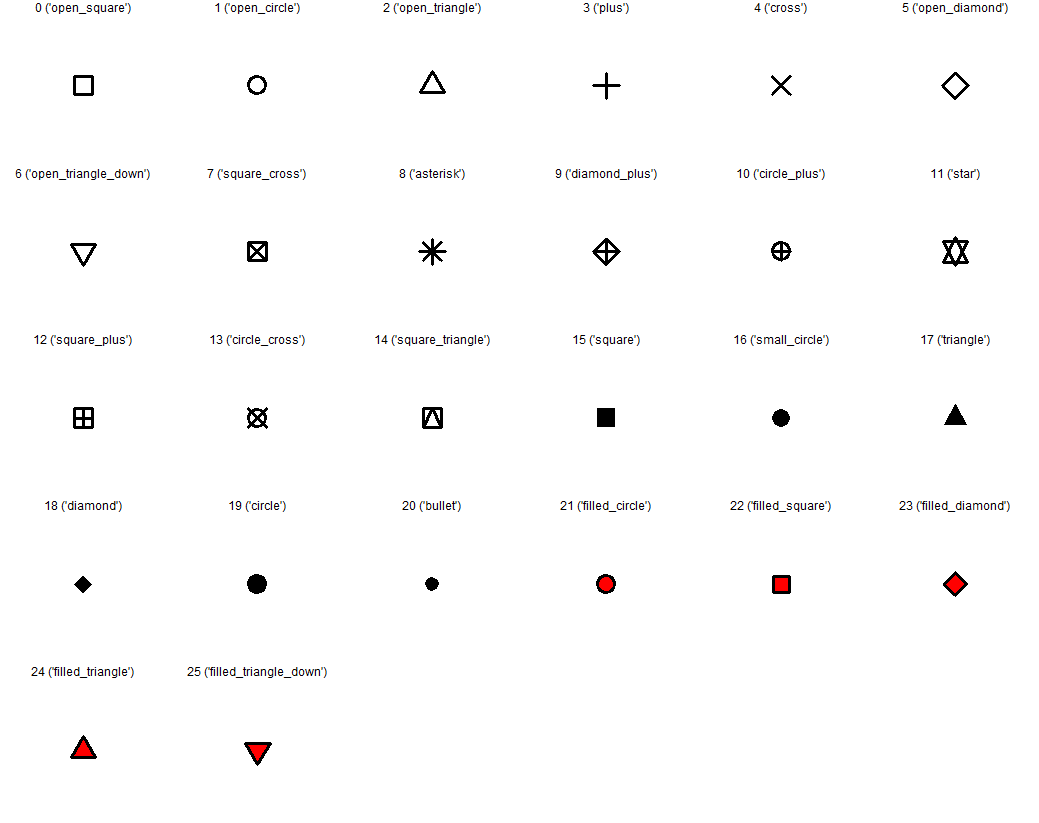###ptoche commented Nov 10, 2017 • edited

 Nice. There is room for disagreement about the relative merits of `filled_circle` and `circle_filled` or `filled.circle` or `circle.filled`. I was under the impression that `x_y` was more for functions, e.g. `geom_boxplot` while `x.y` was more for function arguments, e.g. `outlier.shape`. I could be wrong. Also there are situations where, say if you import a spreadsheet, a `circle filled` gets converted to `circle.filled` so possibly some benefits from having dots. I have no strong opinion. Personally I rarely use shapes outside of 21-26 and have them memorized.

###hturner commented Nov 10, 2017

 I don't have a particular preference for `filled_circle` vs `filled.circle` - I'm happy for a regular contributor to the tidyverse to say which is more consistent with their style. The choice to use "filled" for symbols 21-25 and to use this as prefix rather than a suffix was based on the use in `?pch`, to quote: ``````The following R plotting symbols are can be obtained with pch = 19:25: those with 21:25 can be colored and filled with different colors: col gives the border color and bg the background color (which is "grey" in the figure) - pch = 19: solid circle, - pch = 20: bullet (smaller solid circle, 2/3 the size of 19), - pch = 21: filled circle, - pch = 22: filled square, - pch = 23: filled diamond, - pch = 24: filled triangle point-up, - pch = 25: filled triangle point down. ``````

###hadley commented Nov 14, 2017

 Given that these are strings, I'd prefer `"filled circle"`, and I think the modifiers would be better as suffixes (so when you sort alphabetically so you see related shapes close together), so that would lead to `"circle filled"`.

###daniel-barnett commented Nov 15, 2017

 Thanks for the input, everyone. Here goes the new names. I went with `triangle down filled` (opposed to `triangle filled down`) as I thought it's a bit more logical in that `triangle` and `triangle down` are (slightly) different shapes. ```library(ggplot2) pch_table <- c("0" = "square open", "1" = "circle open", "2" = "triangle open", "3" = "plus", "4" = "cross", "5" = "diamond open", "6" = "triangle down open", "7" = "square cross", "8" = "asterisk", "9" = "diamond plus", "10" = "circle plus", "11" = "star", "12" = "square plus", "13" = "circle cross", "14" = "square triangle", "15" = "square", "16" = "circle small", "17" = "triangle", "18" = "diamond", "19" = "circle", "20" = "bullet", "21" = "circle filled", "22" = "square filled", "23" = "diamond filled", "24" = "triangle filled", "25" = "triangle down filled") df_shapes <- data.frame(shape = 0:25, shape_name = factor(paste0(0:25, " ('", pch_table, "')"))) df_shapes <- df_shapes[order(pch_table),] ggplot(df_shapes, aes(0, 0, shape = shape)) + geom_point(aes(shape = shape), size = 5, fill = 'red', stroke = 2) + scale_shape_identity() + facet_wrap(~reorder(shape_name, shape)) + theme_void() sort(pch_table)```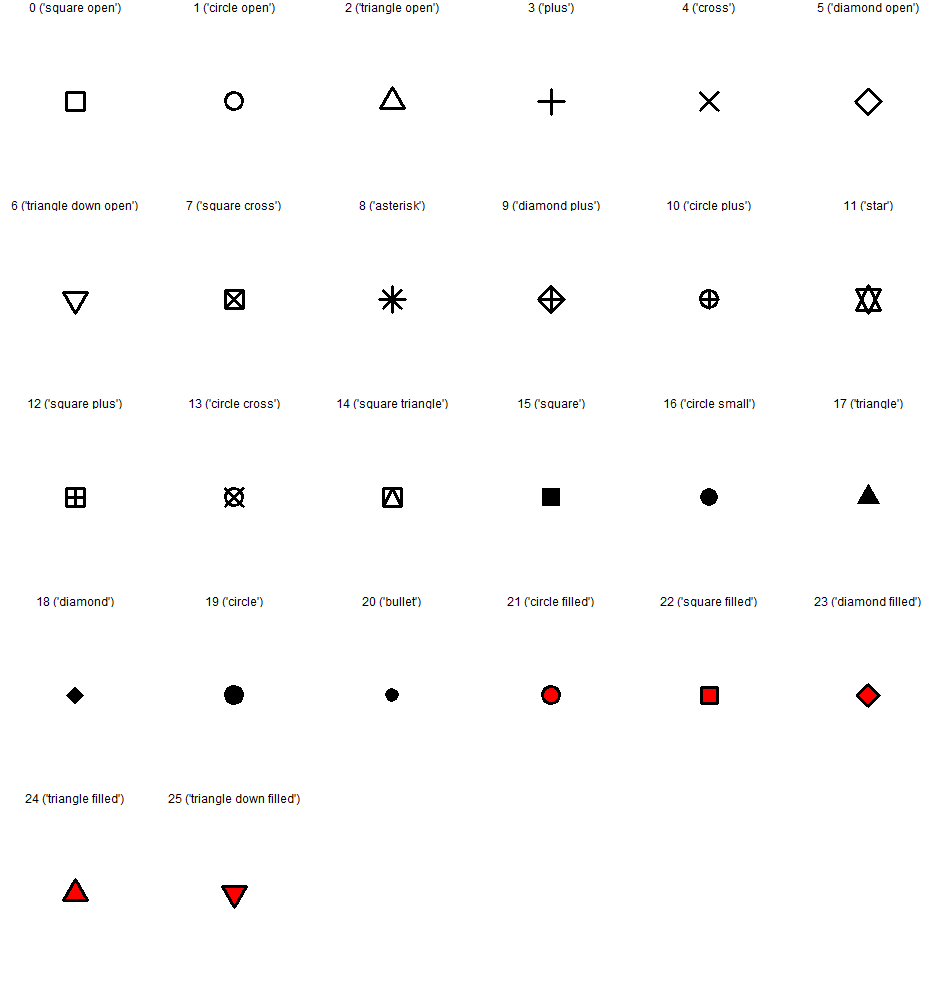`````` 8 20 19 "asterisk" "bullet" "circle" 13 21 1 "circle cross" "circle filled" "circle open" 10 16 4 "circle plus" "circle small" "cross" 18 23 5 "diamond" "diamond filled" "diamond open" 9 3 15 "diamond plus" "plus" "square" 7 22 0 "square cross" "square filled" "square open" 12 14 11 "square plus" "square triangle" "star" 17 25 6 "triangle" "triangle down filled" "triangle down open" 24 2 "triangle filled" "triangle open" ``````

###hadley commented Nov 15, 2017

 Can you please redo that plot alphabetically ordering the shapes? I think your code will be simpler if you flip the names and values.

###ptoche commented Nov 15, 2017 • edited

 like this? ``````pch_table2 <- sort(setNames(names(pch_table), unname(pch_table))) square open circle open "0" "1" circle plus star "10" "11" square plus circle cross "12" "13" square triangle square "14" "15" circle small triangle "16" "17" diamond circle "18" "19" triangle open bullet "2" "20" circle filled square filled "21" "22" diamond filled triangle filled "23" "24" triangle down filled plus "25" "3" cross diamond open "4" "5" triangle down open square cross "6" "7" asterisk diamond plus "8" "9" ``````

###hadley commented Nov 15, 2017

 I meant just redraw the plot alphabetically.

###ptoche commented Nov 15, 2017 • edited

 I'm getting warnings... but the output looks ok: ``````df_shapes <- data.frame(shape = 0:25, shape_name = pch_table, stringsAsFactors = FALSE) df_shapes <- df_shapes[order(df_shapes\$shape_name),] df_shapes\$shape_name <- factor(df_shapes\$shape_name) ggplot(df_shapes, aes(0, 0, shape = shape)) + geom_point(aes(shape = shape), size = 5, fill = 'red', stroke = 2) + scale_shape_identity() + facet_wrap(~ shape_name) + theme_void() ``````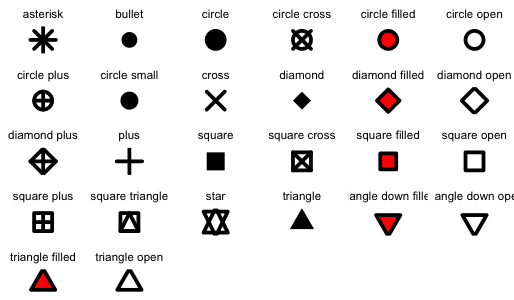###hadley commented Nov 15, 2017

 That's great, thanks! I wonder if `star` should be `triangle up down`? And maybe it would be useful to have manually specified rows and columns? Then you could (e.g.) display the cross and plus variations in the matching columns. This would be useful to help people understand how the shapes are related to one another.

###ptoche commented Nov 16, 2017

 Together with `"triangle down up" <- "triangle up down"` ? and ``````"diamond plus" <- "plus diamond" "square cross" <- "cross square" "square plus" <- "plus square" "square triangle" <- "triangle square" ``````

###ptoche commented Nov 16, 2017 • edited

 do you mean a grid like this one?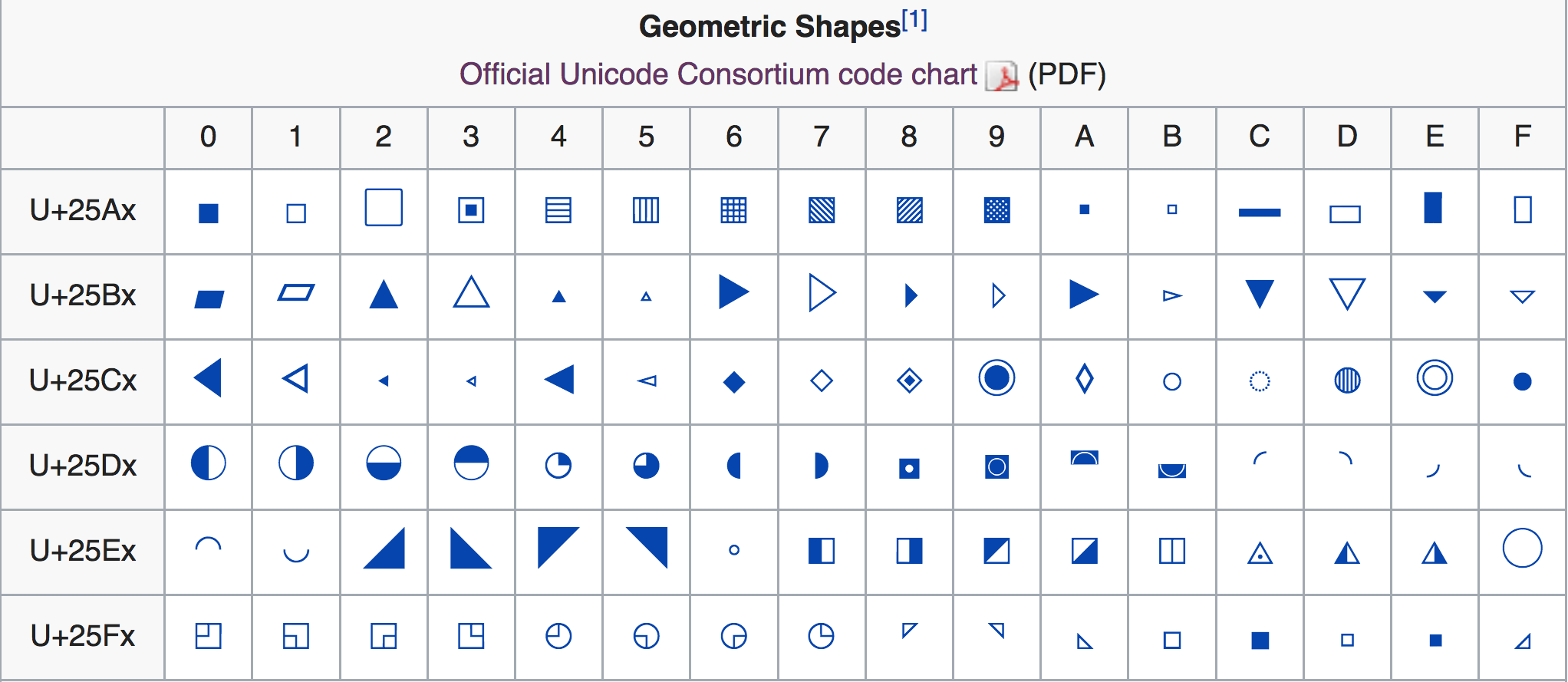or basic shapes along both directions for a triangular matrix effect?

###hturner commented Nov 16, 2017

 Having the modifiers as suffixes makes more sense to me when the shapes are ordered alphabetically. But this leads me to suggest a couple of changes: `"circle tiny"` instead of `"bullet"`? `"nabla"` instead of `"triangle down"`? (https://en.wikipedia.org/wiki/Nabla_symbol). This is a lot shorter to type and if you do sort the shapes alphabetically, the nablas will be separate from the triangles. Another way to separate the two types of triangle when sorting alphabetically would be to use `"triangle rotated"` but this is a lot longer, especially with the filled/open suffix. I still like `"star` as it is short and describes the shape quite well. But `"nabla triangle"`/`"triangle nabla"` also works. I think Hadley's last comment about the plot was to arrange so that the row represented the base shape and the column represented the modifier, e.g. the layout would be (shortened example) `````` [,1] [,2] [,3] [,4] [1,] "plus" "" "" "" [2,] "square" "square cross" "square filled" "square open" [3,] "triangle" "" "triangle filled" "triangle open" `````` In which case alphabetical ordering is less important, but having a one-word name for the base shape is quite useful.

###ptoche commented Nov 16, 2017 • edited

 `"circle tiny"` is more consistent with the naming convention `shape+qualifier`. By this reasoning, `"triangle down"` works better than `"nabla"`.

###hadley commented Nov 16, 2017

 @ptoche I mean a grid where you've carefully arranged the cells by hand so that related symbols appear next to each other. For example, in the previous plot, it would be nice if "triangle filled" and "triangle open" appeared on the far right to align with the filled and open shapes. Similarly, it would be nice if the all the unmodified shapes were arranged in one column. This would make it easier to see the underlying pattern in the shapes.

###baptiste commented Nov 27, 2017

 @hadley the problem is that many shapes belong to more than one family (e.g. both triangle and square),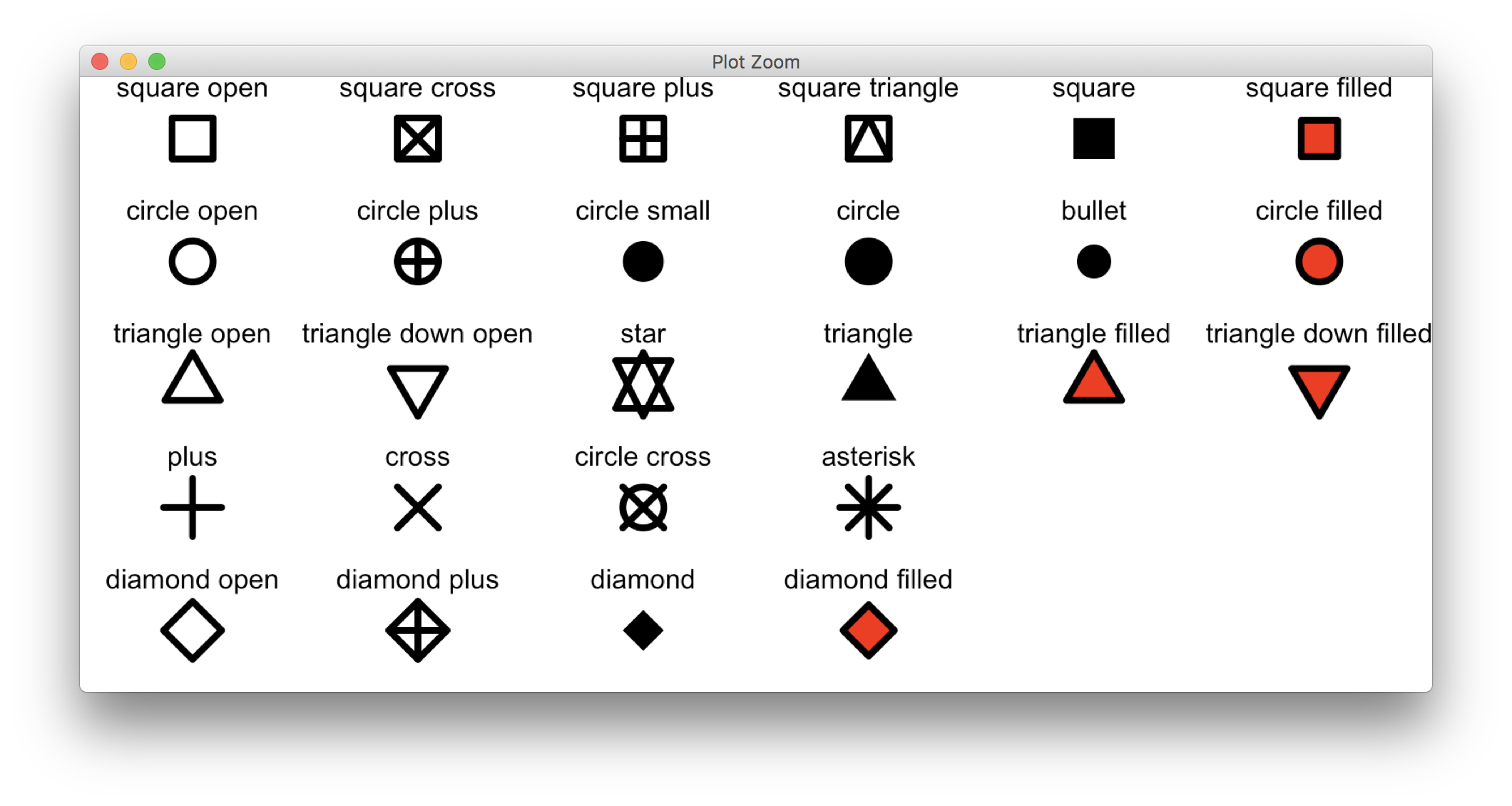###hadley commented Nov 28, 2017

 @baptiste there's no reason for a shape to appear in only one place 😉

###baptiste commented Nov 28, 2017

 There we go again with quantum Venn diagrams ;)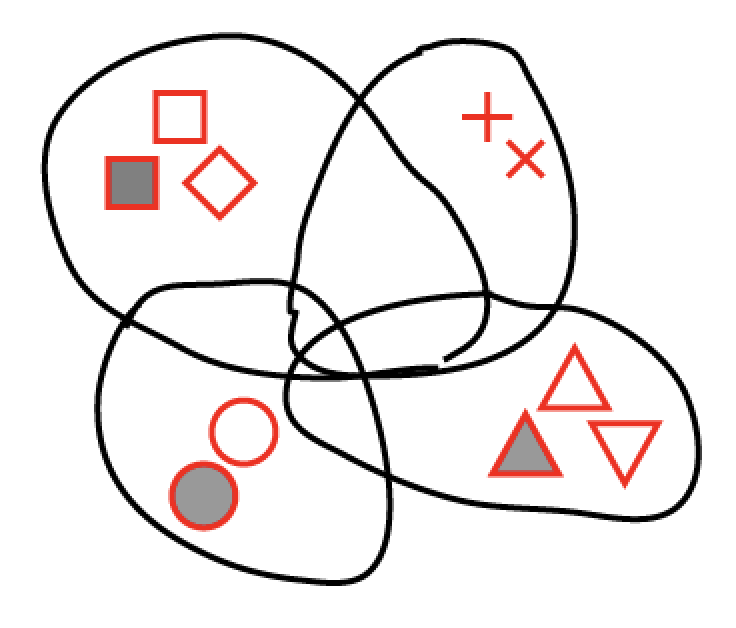I think no matter how one puts it, these shapes are an odd bunch. Years ago I thought it would be nice to get a better set included in the low-level graphics primitives (more consistent, in terms of sizes, attributes, combinations, redundancy, etc.), but that seems unlikely nowadays; instead I hope that something like svg will soon become the only graphics format worth discussing, and all these 'device' quirks will be left behind.

###clauswilke commented May 12, 2018

 Can this issue be closed? It seems to me that this has been addressed with #2338. The following works for me. ```library(ggplot2) mtcars\$am2 <- ifelse(mtcars\$am, "Manual", "Automatic") ggplot(mtcars) + geom_point(aes(hp, cyl, shape = am2)) + scale_shape_manual(values = c("Manual" = "triangle open", "Automatic" = "plus")) ```Created on 2018-05-11 by the reprex package (v0.2.0).

###hadley commented May 12, 2018

 Oops yes

###lock bot commented Nov 8, 2018

 This old issue has been automatically locked. If you believe you have found a related problem, please file a new issue (with reprex) and link to this issue. https://reprex.tidyverse.org/